Firing & Commutation Circuits of SCR - 2

# Firing & Commutation Circuits of SCR - 2 - Notes - Electrical Engineering (EE)

 1 Crore+ students have signed up on EduRev. Have you?

Commutation circuits

• Requirements to be satisfied for the successful turn-off of a SCR
• The turn-off groups as per the General Electric classification
• The operation of the turn-off circuits
• Design of a SCR commutation circuit

A thyristor can be turned ON by applying a positive voltage of about a volt or a current of a few tens of milliamps at the gate-cathode terminals. However, the amplifying gain of this regenerative device being in the order of the 108, the SCR cannot be turned OFF via the gate terminal. It will turn-off only after the anode current is annulled either naturally or using forced commutation techniques. These methods of turn-off do not refer to those cases where the anode current is gradually reduced below Holding Current level manually or through a slow process. Once the SCR is turned ON, it remains ON even after removal of the gate signal, as long as a minimum current, the Holding Current, Ih, is maintained in the main or rectifier circuit.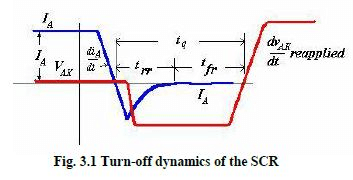In all practical cases, a negative current flows through the device. This current returns to zero only after the reverse recovery time trr, when the SCR is said to have regained its reverse blocking capability. The device can block a forward voltage only after a further tfr, the forward recovery time has elapsed. Consequently, the SCR must continue to be reverse-biased for a minimum of tfr + trr = tq, the rated turn- off time of the device. The external circuit must therefore reverse bias the SCR for a time toff > tq. Subsequently, the reapplied forward biasing voltage must rise at a dv/dt < dv/dt (reapplied) rated. This dv/dt is less than the static counterpart. General Electric has suggested six classification methods for the turn-off techniques generally adopted for the SCR. Others have chosen different classification rules.
SCRs have turn-off times rated between 8 - 50 μsecs. The faster ones are popularly known as 'Inverter grade' and the slower ones as 'Converter grade' SCRs. The latter are available at higher current levels while the faster ones are expectedly costlier.

Classification of forced commutation methods

The six distinct classes by which the SCR can be turned off are:

Class A              Self commutated by a resonating load
Class B              Self commutated by an L-C circuit
Class C             C or L-C switched by another load carrying SCR
Class D             C or L-C switched by an auxiliary SCR

Class E An external pulse source for commutation Class
F AC line commutation
These examples show the classes as choppers. The commutation classes may be used in practice in configurations other than choppers.

Class A, Self commutated by resonating the load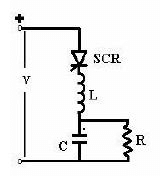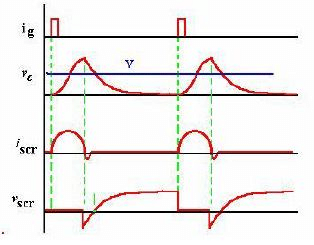Fig. 3.2 A resonant load commutated SCR and the corresponding waveforms

When the SCR is triggered, anode current flows and charges up C with the dot as positive. The L-C-R form a second order under-damped circuit. The current through the SCR builds up and completes a half cycle. The inductor current will then attempt to flow through the SCR in the reverse direction and the SCR will be turned off.
The capacitor voltage is at its peak when the SCR turns off and the capacitor discharges into the resistance in an exponential manner. The SCR is reverse-biased till the capacitor voltages returns to the level of the supply voltage V.

Class B, Self commutated by an L-C circuit

The Capacitor C charges up in the dot as positive before a gate pulse is applied to the SCR. When SCR is triggered, the resulting current has two components.
The constant load current Iload flows through R - L load. This is ensured by the large reactance in series with the load and the freewheeling diode clamping it. A sinusoidal current flows through the resonant L- C circuit to charge-up C with the dot as negative at the end of the half cycle. This current will then reverse and flow through the SCR in opposition to the load current for a small fraction of the negative swing till the total current through the SCR becomes zero. The SCR will turn off when the resonant–circuit (reverse) current is just greater than the load current.
The SCR is turned off if the SCR remains reversed biased for tq > toff, and the rate of rise of the reapplied voltage < the rated value.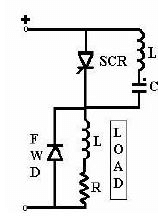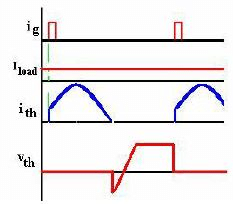Problem #1
A Class B turn-off circuit commutates an SCR. The load current is constant at 10 Amps. Dimension the commutating components L and C. The supply voltage is 100VDC.

Soln # 1
The commutating capacitor is charged to the supply voltage = 100 V
The peak resonant current is,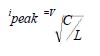Assuming,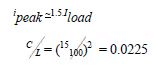The SCR commutates when the total current through it reaches zero.This corresponds to 0.73 rads after the zero crossing of the resonant current. The capacitor voltage at that instant is 75 volts. After the SCR turns off, the capacitor is charged linearly by the load current.

If the SCR is to commutate at twice this load current, for a rated "Inverter grade' SCR turn-off time of 20 μsecs,

It can be observed that if the peak of the commutating current is just equal to the load current, the turn-off time would be zero as the capacitor would not be able to impress any negative voltage on the SCR.

Class C, C or L-C switched by another load–carrying SCR

This configuration has two SCRs. One of them may be the main SCR and the other auxiliary. Both may be load current carrying main SCRs. The configuration may have four SCRs with the load across the capacitor, with the integral converter supplied from a current source. Assume SCR2 is conducting. C then charges up in the polarity shown. When SCR1 is triggered, C is switched across SCR2 via SCR1 and the discharge current of C opposes the flow of load current in SCR2.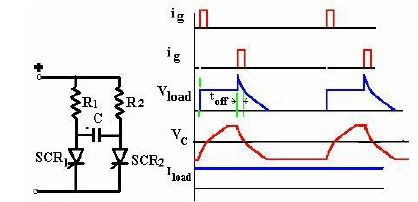Fig. 3.4 Class C turn-off, SCR switched off by another load-carring SCR

Class D, L-C or C switched by an auxiliary SCR

Example 1

The circuit shown in Figure 3.3 (Class C) can be converted to Class D if the load current is carried by only one of the SCR’s, the other acting as an auxiliary turn-off SCR. The auxiliary SCR would have a resistor in its anode lead of say ten times the load resistance.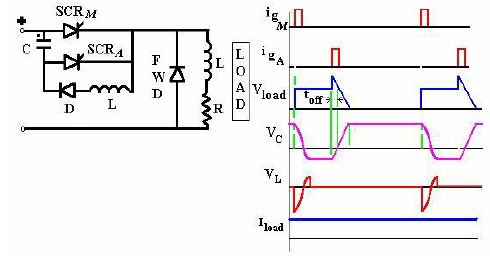Fig. 3.5 Class D turn-off. Class D commutation by a C (or LC) switched by an Auxiliary SCR.

Example 2

SCRA must be triggered first in order to charge the upper terminal of the capacitor as positive. As soon as C is charged to the supply voltage, SCRA will turn off. If there is substantial inductance in the input lines, the capacitor may charge to voltages in excess of the supply voltage. This extra voltage would discharge through the diode-inductor-load circuit.
When SCRM is triggered the current flows in two paths: Load current flows through the load and the commutating current flows through C- SCRM -L-D network. The charge on C is reversed and held at that level by the diode D. When SCRA is re-triggered, the voltage across C appears across SCRM via SCRA and SCRM is turned off. If the load carries a constant current as in Fig. 3.4, the capacitor again charges linearly to the dot as positive.

Problem # 2

A Class D turn-off circuit has a commutating capacitor of 10 μF. The load consists of a clamped inductive load such that the load current is reasonably constant at 25 amperes. The 'Inverter grade' SCR has a turn-off time of 12 μsecs. Determine whether the SCR will be satisfactorily commutated. Also dimension the commutating inductor. The supply voltage is 220 VDC.

Soln # 2

The capacitor is initially charged to the supply voltage 220 V at the end of the conduction period of SCRA.
When SCRM is triggered, the 25 Amps load current and the L-C ringing current flows through it. Peak current through SCR is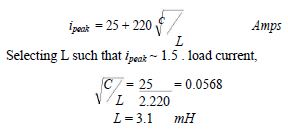Assuming that the capacitor charges to 70% of its original charge because of losses in the C- SCRM -L-D network, and it charges linearly when SCRA is again triggered,

q =1540 / 25 =61.6 μsec s

The SCR can therefore be successfully commutated.

The maximum current that can be commutated with the given Capacitor at the 220 V supply voltage is

For the 25 Amps load current the capacitor just enough would have a rating of

C = Iload .tq /(0.7.220) = (25.12) /154 =1.95 ≈ 2.0 μF

If the supply voltage is reduced by a factor K, the required capacitor rating increases by the same factor K for the same load current.

Class E – External pulse source for commutation

The transformer is designed with sufficient iron and air gap so as not to saturate. It is capable of carrying the load current with a small voltage drop compared with the supply voltage.
When SCR1 is triggered, current flows through the load and pulse transformer. To turn SCR1 off a positive pulse is applied to the cathode of the SCR from an external pulse generator via the pulse transformer. The capacitor C is only charged to about 1 volt and for the duration of the turn-off pulse it can be considered to have zero impedance. Thus the pulse from the transformer reverses the voltage across the SCR, and it supplies the reverse recovery current and holds the voltage negative for the required turn-off time.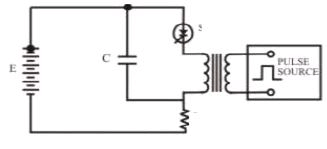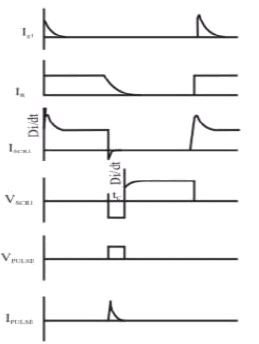Fig. 3.6 Class E, External pulse commutation

Class F, AC line commutated

If the supply is an alternating voltage, load current will flow during the positive half cycle. With a highly inductive load, the current may remain continuous for some time till the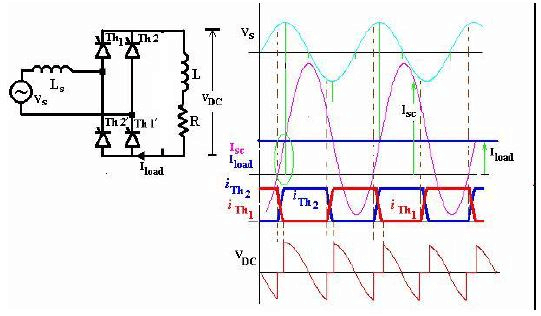Fig. 3.7 Class F, natural commutation by supply voltage

energy trapped in the load inductance is dissipated. During the negative half cycle, therefore, the SCR will turn off when the load current becomes zero 'naturally'. The negative polarity of the voltage appearing across the outgoing SCR turns it off if the voltage persists for the rated turn-off period of the device. The duration of the half cycle must be definitely longer than the turn-off time of the SCR.

The rectifier in Fig.3.6 is supplied from an single phase AC supply. The commutation process involved here is representative of that in a three phase converter. The converter has an input inductance Ls arising manly out of the leakage reactance of the supply transformer. Initially, SCRs Th1 and Th1' are considered to be conducting. The triggering angle for the converter is around 600. The converter is operating in the continuous conduction mode aided by the highly-inductive load.

When the incoming SCRs, Th2 and Th2' are triggered, the current through the incoming devices cannot rise instantaneously to the load current level. A circulating current Isc builds up in the short-circuited path including the supply voltage, Vs-Ls-Th1'- Th2 and Vs- Ls-Th2'-Th1 paths. This current can be described by: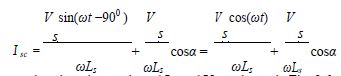where α the triggering angle and Isc and Vs as shown in Fig. 3.6.

This expression is obtained with the simplifying assumption that the input inductance contains no resistances. When the current rises in the incoming SCRs, which in the outgoing ones fall such that the total current remains constant at the load current level. When the current in the incoming ones reach load current level, the turn-off process of the outgoing ones is initiated. The reverse biasing voltage of these SCRs must continue till they reach their forward blocking state. As is evident from the above expression, the overlap period is a function of the triggering angle. It is lowest when α ~ 900. These SCRs being 'Converter grade', they have a larger turn-off time requirement of about 30-50 μsecs.

The period when both the devices conduct is known as the 'overlap period'. Since all SCRs are in conduction, the output voltage for this period is zero. If the 'fully-controlled' converter in Fig. 3.7 is used as an inverter with triggering angles > 900, the converter triggering can be delayed till the 'margin angle' which includes the overlap angle and the turn-off time of the SCR - both dependent on the supply voltages.

Rate of rise of forward voltage, dv/dt

The junctions of any semiconductor exhibit some unavoidable capacitance. A changing voltage impressed on this junction capacitance results in a current, I = C dv/dt. If this current is sufficiently large a regenerative action may occur causing the SCR to switch to the on state. This regenerative action is similar to that which occurs when gate current is injected. The critical rate of rise of off-state voltage is defined as the maximum value of rate of rise of forward voltage which may cause switching from the off-state to the on-state.

Since dv/dt turn-on is non-destructive, this phenomenon creates no problem in applications in which occasional false turn -on does not result in a harmful affect at the load. Heater application is one such case. However, at large currents where dv/dt turn-on is accompanied by partial turn-on of the device area a high di/dt occurs which then may be destructive.

The majority of inverter applications, however, would result in circuit malfunction due to dv/dt turn-on. One solution to this problem is to reduce the dv/dt imposed by the circuit to a value less than the critical dv/dt of the SCR being used. This is accomplished by the use of a circuit similar to those in Figure 3.8 to suppress excessive rate of rise of anode voltage. Z represents load impedance and circuit impedance. Variations of the basic circuit is also shown where the section of the network shown replaces the SCR and the R-C basic snubber.

Since circuit impedances are not usually well defined for a particular application, the values of R and C are often determined by experimental optimization. A technique can be used to simplify snubber circuit design by the use of nomographs which enable the circuit designer to select an optimized R-C snubber for a particular set of circuit operating conditions.

Another solution to the dv/dt turn-on problem is to use an SCR with higher dv/dt turn-on problem is to use an SCR with higher dv/dt capability. This can be done by selecting an SCR designed specially for high dv/dt applications, as indicated by the specification sheet. Emitter shorting is a manufacturing technique used to accomplish high dv/dt capability.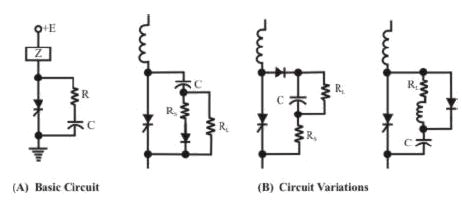Fig. 3.8 dv/dt supression circuits

Questions

• 1 For a Class D turn-off SCR, the load consists of a resistance only. If the supply voltage and SCR turn-off ratings are as in Problem # 1 calculate the required value of the commutating capacitor.

Ans: (Hints): The capacitor would now charge in an exponential manner. The time it takes to discharge from its reverse charged state once SCRA is triggered is the circuit turn-off time which must be in excess of the rated 12 μsecs.

• 2 For a Class F converter, will the overlap period rise with the leakage inductance of the converter? What happens to the output voltage?

Ans: Yes. The overlap time is directly related to the commutating inductance. The output voltage decreases. In fact, this inductor limits the maximum output current of the converter. The input current maximum would be as for a shorted network with the leakage inductance only present.

• 3 Can the output DC voltage be controlled in the above circuits?

Ans: Yes. Most of the above circuits are also called 'forced commutated' DC-DC chopper circuits.

The document Firing & Commutation Circuits of SCR - 2 - Notes - Electrical Engineering (EE) is a part of Electrical Engineering (EE) category.
All you need of Electrical Engineering (EE) at this link: Electrical Engineering (EE)

Track your progress, build streaks, highlight & save important lessons and more!

,

,

,

,

,

,

,

,

,

,

,

,

,

,

,

,

,

,

,

,

,

;